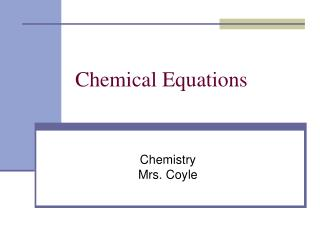DownloadDownload PresentationChemical Equations

# Chemical Equations

Télécharger la présentation## Chemical Equations

- - - - - - - - - - - - - - - - - - - - - - - - - - - E N D - - - - - - - - - - - - - - - - - - - - - - - - - - -
##### Presentation Transcript

1. Chemical Equations ChemistryMrs. Coyle

2. Chemical Equations: represent chemical reactions • Word Equations • Skeleton Chemical Equations • Balanced Chemical Equations

3. Word Equations: show the names of the reactants and the products Reactants  Products Methane + Oxygen  Carbon Dioxide + Water • The arrow (yield sign) and indicates that a reaction took place.

4. Skeleton Chemical Equations The formulas of the reactants and products are written without indicating their relative amounts. Example: CH4+ O2 CO2 +H2O

5. Balanced Chemical Equations • The chemical formulas and the relative amounts of the reactants and products are written. Example: CH4 + 2O2 CO2 + 2H2O • The coefficients indicate the relative amounts of each substance. • Equal number of atoms of each element must appear on both sides of the balanced equation.

6. Why does a chemical reaction have to be balanced? • In any chemical or physical change, mass is neither created or destroyed • Mass is CONSTANT • Law of Conservation of Mass (Antoine Lavoisier)

7. Combustion of MethaneCH4 + 2O2 CO2 + 2H2O Atoms are rearranged!

8. Example: Decomposition of hydrogen peroxide in the presence of a catalyst. • Word equation: hydrogen peroxide  water + oxygen

9. Example: Decomposition of hydrogen peroxide in the presence of a catalyst. • Skeleton equation: H2O2  H2O + O2

10. Example: Decomposition of hydrogen peroxide in the presence of a catalyst. • Balanced Equation: 2H2O2  2H2O + O2

11. Example: Decomposition of hydrogen peroxide in the presence of a catalyst. Balanced equation showing • the catalyst (MnO2) • the state of the reactants and products: MnO2 2H2O2(l) 2H2O (l) + O2 (g)